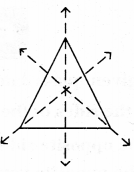# AP Board 6th Class Maths Solutions Chapter 9 2D-3D Shapes Ex 9.3

AP State Syllabus AP Board 6th Class Maths Solutions Chapter 9 2D-3D Shapes Ex 9.3 Textbook Questions and Answers.

## AP State Syllabus 6th Class Maths Solutions 9th Lesson 2D-3D Shapes Ex 9.3Question 1.
i) What are the sides of the given quadrilateral?
ii) What is the opposite side of $$\overline{\mathrm{AB}}$$ ?
iii) What is the opposite vertex of B?
iv) What is the opposite angle of ∠C ?
v) How many pairs of adjacent angles are there? What are they?
vi) How many pairs of opposite angles are there? What are they?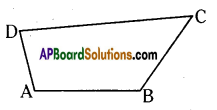Solution:
i) It has four sides. They are $$\overline{\mathrm{AB}}, \overline{\mathrm{BC}}, \overline{\mathrm{CD}} \text { and } \overline{\mathrm{AD}}$$
ii) Opposite side of $$\overline{\mathrm{AB}}$$ is $$\overline{\mathrm{DC}}$$
iii) Opposite vertex to B is D.
iv) Opposite angle of ∠C is ∠BAD (or) ∠A.
v) It has four pair of adjacent angles are there. They are :
Adjacent angles of ∠A are ∠B and ∠D.
Adjacent angles of ∠B are ∠A and ∠C.
Adjacent angles of ∠C are ∠B and ∠D.
Adjacent angles of ∠D are ∠A and ∠C.
vi) It has two pairs of opposite angles are there. They are ∠A and ∠C; ∠B and ∠D.

Question 2.
Find the number of lines of symmetry in the following.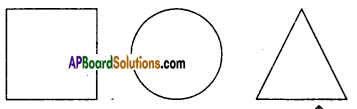Solution:
i) Given adjacent figure is a square.
Number of lines of symmetry to a square are 4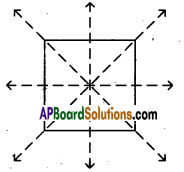ii) Given adjacent figure is a circle.
Number of lines of symmetry to a circle are infinite (many).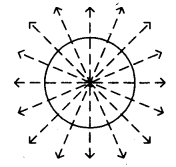iii) Given adjacent figure is a triangle.
Number of lines of symmetry to the triangle are three.
(If it is an equilateral triangle).